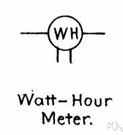watt-hour

(redirected from Kilowatthour)
Also found in: Thesaurus, Encyclopedia.
Related to Kilowatthour: KWHR, Megawatt hour

watt-hour

(wŏt′our′)
n. Abbr. WH or W-hr
A unit of energy, especially electrical energy, equal to the work done by one watt acting for one hour and equivalent to 3,600 joules.

watt-hour

n
(Units) a unit of energy equal to a power of one watt operating for one hour. 1 watt-hour equals 3600 joules

or watt′hour`,

n.
a unit of energy equal to the energy of one watt operating for one hour, equivalent to 3600 joules. Abbr.: Wh

watt-hour

A unit of power, especially electrical power, equal to the work done by one watt acting for one hour. It is equivalent to 3,600 joules.
ThesaurusAntonymsRelated WordsSynonymsLegend:
 Noun 1watt-hour - a unit of energy equal to the power of one watt operating for one hourenergy unit, heat unit, work unit - a unit of measurement for workB.T.U., Board of Trade unit, kilowatt hour, kW-hr - a unit of energy equal to the work done by a power of 1000 watts operating for one hour
References in periodicals archive ?
5 cents per kilowatthour (kWh) for the electricity they use.
0781 per kilowatthour (kWh), thereby bringing it down to PhP8.
cents per kilowatthour, compared to 5-8 cents for onshore wind.
As a result, the technology may allow for PV system installation costs of US\$3 per watt, with electricity production costs of 8 to 10 cents per kilowatthour.
On the other hand, the report estimates that use of CCS would raise electricity costs by 1 to 5 cents per kilowatthour, depending on the fuel, location, and other variables, even with new generating plants that incorporated CCS technology from the start.
32 per kilowatthour to cover maintenance costs for generators.
20 per kilowatthour (kwh), Dasureco's consumers will now have to pay P9.
4 cents per kilowatthour (2003 dollars) in 2003 to a low of 6.

Site: Follow: Share:
Open / Close